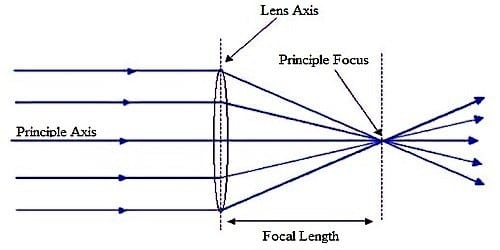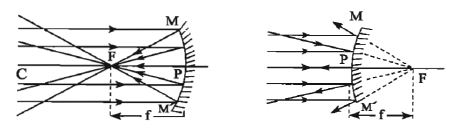# What is Principal Focus of the Mirror?

A beam of rays adjacent and parallel to the principal axis being incident on a spherical mirror converges at a point on the principal axis (in case of concave mirror) or appears to be diverging from a point on the principal axis (in case of convex mirror) after reflection, then this point is called the principal focus of the mirror.

The principal focus is the focus for a beam of incident rays parallel to the axis.

The principal focus of a spherical mirror is a point on the principal axis of the spherical mirror at which, the light rays which are parallel to the principal axis essentially converge (meet) or emerge to deviate after reflection.

Mirrors work by altering the direction that light is moving. The principle focuses on the convex and concave lens are contradictory in direction. To make an image, we can trace a few rays that the light makes and see what happens to them. The focal length of the convex lens is positive and for the concave lens, it is negative.Focal plane: The plane imagined that passes through the principal focus and perpendicular to the principal axis is called the focal plane.

Focal length: If you were to take a concave mirror and shine a whole lot of rays of light straight at it (all parallel to each other), then all of those beams would be reflected by the mirror, and they’d all congregate at one point. That point is what’s called the focal point (or the principal focus). The distance between the pole and the principal focus of a spherical mirror is called the focal length of the mirror. In figure (1) and (2), PF is the focal length. In a spherical mirror, the focal length is half of the radius of curvature. Therefore, f = r/2.

The principal focus of Concave Mirror:

In a concave mirror, the light gets reflected towards the center. A number of rays which falls parallel to the principal axis on a concave mirror meet at a point on the principal axis after getting reflected. Light rays that are parallel to the principal axis of a concave mirror converge at a particular point on its principal axis after reflecting from the mirror.  This is known as the principal focus of a concave mirror. This point is known as the principal focus of the concave mirror.

The principal focus of Convex Mirror:

In a convex mirror, the light moves away. A number of rays which falls parallel to the principal axis after getting reflected, the rays appears to come from a point on the principal axis.